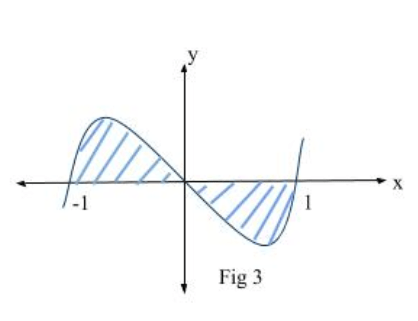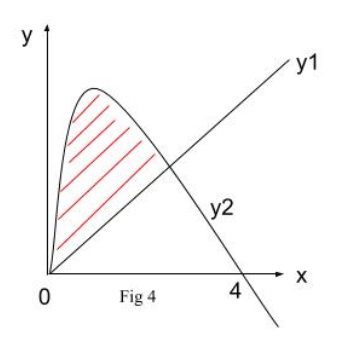# Area under a curve

#### Summary

• Area under a curve can only be calculated if the integral is definite. It must have limits.
• We must be aware of the three common scenarios when working out the areas under the curves:
1. Find area between a curve, the x axis and the line x = a and x = b.
2. Find the area of curve under the x axis.
3. Find the area between a curve and a straight line.

Area under a curve can be calculated by integrating definite integrals.

Definite Integration is when we integrate a function between defined limits. The function must have an upper limit and a lower limit. It is defined as:$\int _{ a }^{ b }{ f^{ ' }\left( x \right) dx } \quad =\quad f\left( b \right) \quad -\quad f\left( a \right)$

Where$f^{ ' }\left( x \right)$ is the derived function of f throughout the interval (a, b).

There are 3 different types of cases which we should be able to identify and solve when integrating a function to find area under curves:

1. First case (Fig 1) is to find area between a curve, the x axis and the line x = a and x = b.

Now we know$Area\quad =\quad \int _{ a }^{ b }{ y } \quad dx$ where$f\left( x \right)$ is the equation of the curve.$A\quad =\quad \int _{ a }^{ b }{ f(x) } \quad dx$

Definite integral of f(x) between x = a & x = b

Example #1

Q. Find the area of the region R bounded by the curve with equation

y = (4 – x)(x + 2) and the positive x and y axis. Graph is shown in Fig 2.Solution:

We first find the coordinates for the shaded region. We know when the curve intersects the y axis, x = 0 & when the curve intersects the x axis, y = 0.

Therefore by putting x = 0 in the equation of the curve we get the y intersect which is equal to 8. Similarly by putting y = 0 we get the x intersect which is equal to -2 and 4 (as the curve intersects both the +x axis and the -x axis).

As we are integrating wrt x, limits would be 0 & 4.

Hence, the area of R is given by:$A\quad =\quad \int _{ 0 }^{ 4 }{ (4\quad -\quad x)(x\quad +\quad 2)\quad dx }$$A\quad =\quad \int _{ 0 }^{ 4 }{ (8\quad +\quad 2x\quad -\quad { x }^{ 2 })\quad dx }$$A\quad =\quad { \left[ 8x\quad +\quad { x }^{ 2 }\quad -\quad \frac { { x }^{ 3 } }{ 3 } \right] }_{ 0 }^{ 4 }$$A\quad =\quad \left( 32\quad +\quad 16\quad -\quad \frac { 64 }{ 3 } \right) \quad -\quad \left( 0 \right)$$A\quad =\quad 26.67$

2. Secondly, find the area of curve under the x axis.

All the above examples had the shaded region above the x axis. However there may be cases where the area between the curve and the x axis lie below the x axis, then integrating the function will give us a negative answer.

This could really affect your answer in cases where you find an area which straddles the x axis as shown in Fig 3.Example #2

Q. Find the area of the shaded region in Fig 3 bounded by the curve with equation$y\quad =\quad (x\quad +\quad 1)(x\quad -\quad 1)\quad =\quad { x }^{ 3 }\quad -\quad x$.

Notice that if we try and solve for the total shaded region from -1 to 1 we will end up getting zero. As both the areas are equal but have opposite signs.$\int _{ -1 }^{ 1 }{ \left( { x }^{ 3 }\quad -\quad x \right) } dx\quad =\quad { \left[ \frac { { x }^{ 4 } }{ 4 } \quad -\quad \frac { { x }^{ 2 } }{ 2 } \right] }_{ -1 }^{ 1 }$$=\quad \left( \frac { 1 }{ 4 } \quad -\quad \frac { 1 }{ 2 } \right) \quad -\quad \left( \frac { 1 }{ 4 } \quad -\quad \frac { 1 }{ 2 } \right)$

Ans:$=\quad 0\quad (this\quad is\quad not\quad correct)$

We must divide the limits of the whole shaded region in two parts i.e from (-1 to 0) and (0 to 1 ). We integrate the equation separately for both limits, then ignore the negative sign on the area of the part which is below the x axis as the sign is only indicating the position of the curve. Lastly we add the two areas which will give us the total area of the shaded region. This is shown below:$\int _{ 0 }^{ 1 }{ \left( { x }^{ 3 }\quad -\quad x \right) } dx\quad =\quad { \left[ \frac { { x }^{ 4 } }{ 4 } \quad -\quad \frac { { x }^{ 2 } }{ 2 } \right] }_{ 0 }^{ 1 }$$=\quad \left( \frac { 1 }{ 4 } \quad -\quad \frac { 1 }{ 2 } \right) \quad -\quad \left( 0 \right)$$=\quad -\frac { 1 }{ 4 }$$\int _{ -1 }^{ 0 }{ \left( { x }^{ 3 }\quad -\quad x \right) } dx\quad =\quad { \left[ \frac { { x }^{ 4 } }{ 4 } \quad -\quad \frac { { x }^{ 2 } }{ 2 } \right] }_{ -1 }^{ 0 }$$=\quad -\left( \frac { 1 }{ 4 } \quad -\quad \frac { 1 }{ 2 } \right)$$=\quad \frac { 1 }{ 4 }$

Area of the shaded region is$=\quad \frac { 1 }{ 4 } \quad +\quad \frac { 1 }{ 4 } \quad =\quad \frac { 1 }{ 2 }$

3. Last case is find the area between a curve and a straight line.

The area between a line (equation y1) and a curve (equation y2) is given by$Area\quad =\quad \int _{ a }^{ b }{ (y1\quad -\quad y2) } dx$ which could also be written as$Area\quad =\quad \int _{ a }^{ b }{ y1dx\quad -\quad } \int _{ a }^{ b }{ y2dx }$

Example #3

Diagram (Fig 4) shows a curve with equation y2 = x(4 – x) and the line with equation y1 = x.To find the area of the region bounded by the curve and the line, we first find the point of intersection between the curve and the line.
We do this by equating both the equations:$x(4\quad -\quad x)\quad =\quad x$$4x\quad -\quad { x }^{ 2 }\quad =\quad x$

Take$4x\quad -\quad { x }^{ 2 }$ to the other side of the equal to sign.${ x }^{ 2 }\quad -\quad 3x\quad =\quad 0$$x(x\quad -\quad 3)\quad =\quad 0$

x = 0 or x = 3

Find y coordinates by substituting x back in one of the equations. The line y1 = x is the simplest one.
Therefore, the line cuts the curve at (0,0) & (3,3).
Next we use the formula and integrate the expression.$Area\quad =\quad \int _{ 0 }^{ 3 }{ \left[ x(4\quad -\quad x)\quad -\quad x \right] dx }$$=\quad \int _{ 0 }^{ 3 }{ (3x\quad -\quad { x }^{ 2 }) } dx$$=\quad { \left[ \frac { 3 }{ 2 } { x }^{ 2 }\quad -\quad \frac { { x }^{ 3 } }{ 3 } \right] }_{ 0 }^{ 3 }$$=\quad (\frac { 27 }{ 9 } \quad -\quad 9)\quad -\quad (0)\quad =\quad 4.5$

##### Reference
1. Core Mathematics 2, Edexcel AS and A Level Modular Mathematics C2